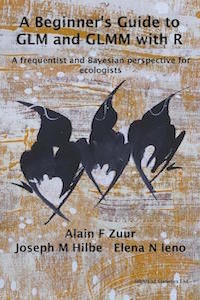www.highstat.com
Highland Statistics Ltd

Latest news

Coming in September 2022:

• Time Series Analysis using Regression Techniques.
• Introduction to GAM and GAMM (frequentist).

See highstat.com for details.# Beginner's Guide to GLM and GLMM with R (2013). Zuur AF, Hilbe JM and Ieno EN

This book presents generalized linear models (GLM) and generalized linear mixed models (GLMM) based on both frequency-based and Bayesian concepts.

Using ecological data from real-world studies, the text introduces the reader to the basics of GLM and mixed effects models, with demonstrations of Gaussian, binomial, gamma, Poisson, negative binomial regression, beta and beta-binomial GLMs and GLMMs.

Visit the website of this book to download data, R code, the table of content, and information how to purchase this book.

• Default
• Title
• Date
• Random